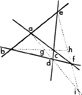Jump to word:

Phrases starting with the letter: A B C D E F G H I J K L M N O P Q R S T U V W X Y Z

Previous word: quadriga
Next word: quadrilingual

# Definition of: quadrilateral

(kwod′rə·latər·əl) adjective
Formed or bounded by four lines; four-sided.
noun

1. Geom. a A figure bounded by four straight lines terminated at four angles. b A figure formed of four infinite straight lines, having six intersections.
2. A space or area defended by four enclosing fortresses. [<L quadrilaterus <quattuor four + latus, lateris side]QUADRILATERAL
abdc. Quadrilateral.
ad., bc. Diagonals.
ebfc. Quadrilateral.
a., b., c., d., f. Vertices.
ai., bh., ei. Diagonals.
g., hh., i. Centers.

### 'quadrilateral' used in domains:

quadrilateralquest.com
quadrilateralstudios.com
lambertquadrilateral.com
quadrilateralperspective.com
quadrilaterals-inc.com
quadrilateralairhockey.com

### Statistical data

"quadrilateral" has the frequency of use of 0.0001% on en.wikipedia.org.

Phrases starting with the letter: A B C D E F G H I J K L M N O P Q R S T U V W X Y Z

## User Contributions:

#### Comment about this word, ask questions, or add new information about this topic: